#### Chapter 19 Surface Area and Volume of a Right Circular Cylinder R.D. Sharma Solutions for Class 9th Exercise 19.2

Exercise 19.2

1. A soft drink is available in two packs-(i) a tin can with a rectangular base of length 5 cm and width 4 cm, having a height of 15 cm and (ii) a plastic cylinder with circular base diameter 7 cm and height 10 cm. Which container has greater capacity and by how much ?

Solution2. The pillars of a temple are cylindrically shaped. If each pillar has a circular base of radius 20 cm and height 10 m. How much concrete mixture would be required to build 14 such pillars ?

Solution3. The inner diameter of a cylindrical wooden pipe is 24 cm and its outer diameter is 28 cm. The length of the pipe is 35 cm. Find the mass of the pipe, if 1 cm3 of wood has a mass of 0.6 gm.

Solution4. If the lateral surface of a cylinder is 94.2  cm2 and its height is 5 cm, find:
(ii) volume of the cylinder [use π=3.14]

Solution5. The capacity of a closed cylindrical vessel of height 1 m is 15.4 litres. How many square metres of metal sheet would be needed to make it ?

Solution6. A patient in a hospital is given soup daily in a cylindrical bowl of diameter 7 cm. If the bowl is filled with soup to a height of 4 cm, how much soup the hospital has to prepare daily to server 250 patients ?

Solution7. A hollow garden roller, 63 cm wide with a girth of 440 cm, is made of 4 cm thick iron. Find the volume of the iron.

Solution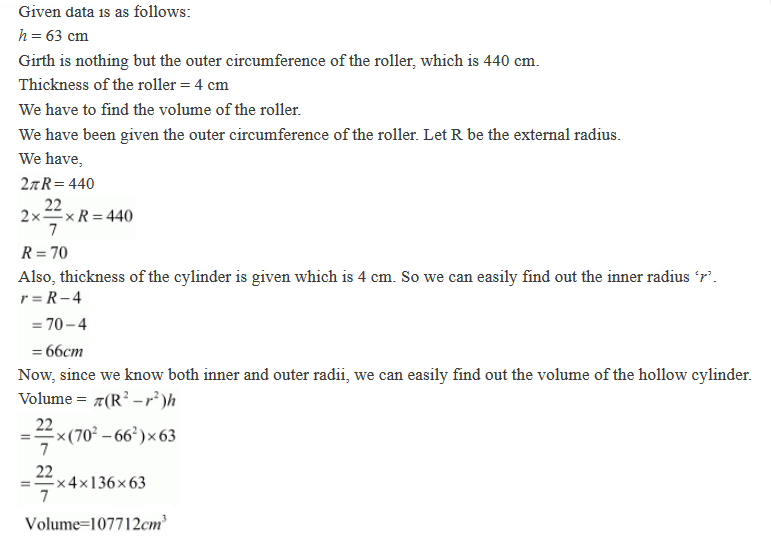8. A solid cylinder has a total surface area of 231 cm2. Its curved surface area is 2/3 of the total surface area. Find the volume of the cylinder.

Solution9. The cost of painting the total outside surface of a closed cylindrical oil tank at 50 paise per square decimetre is Rs 198. The height of the tank is 6 times the radius of the base of the tank. Find the volume corrected to 2 decimal places.

Solution10. The radii of two cylinders are in the ratio 2 : 3 and their heights are in the ratio 5 : 3. Calculate the ratio of their volumes and the ratio of their curved surfaces.

Solution11. The ratio between the curved surface area and the total surface area of a right circular cylinder is 1 : 2. Find the volume of the cylinder, if its total surface area is 616 cm2.

Solution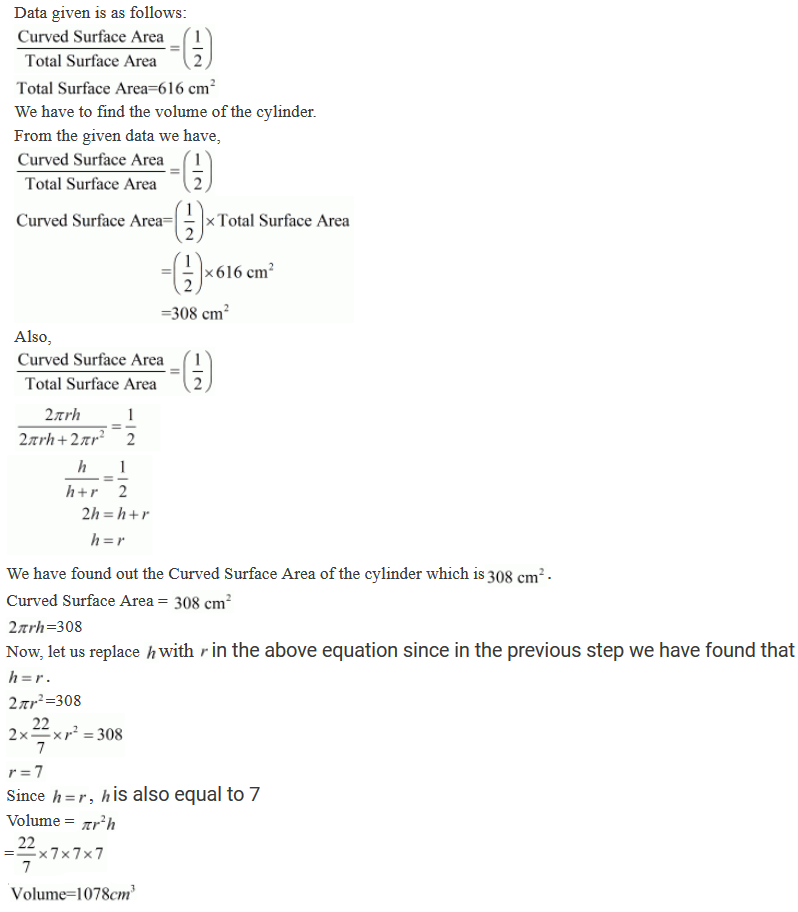12. The curved surface area of a cylinder is 1320 cm2 and its base had diameter 21 cm. Find the height and the volume of the cylinder.

Solution13. The ratio between the radius of the base and the height of a cylinder is 2 : 3. find the total surface area of the cylinder, it its volume is 1617 cm3.

Solution14. A rectangular sheet of paper, 44 cm × 20 cm, is rolled along its length of form a cylinder. Find the volume of the cylinder so formed.

Solution15. The curved surface area of a cylindrical pillar is 264 m2 and its volume is 924 m3. Find the diameter and the height of the pillar.

Solution16. Two circular cylinders of equal volumes have their heights in the ratio 1 : 2. Find the ratio of their radii.

Solution17. The height of a right circular cylinder is 10.5 m. Three times the sum of the areas of its two circular faces is twice the area of the curved surface. Find the volume of the cylinder.

Solution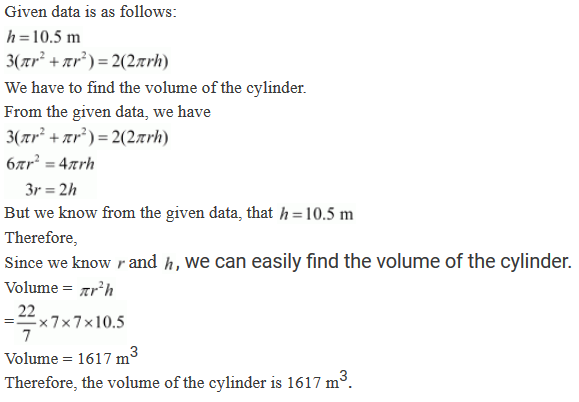18. How many cubic metres of earth must be dugout to sink a well 21 m deep and 6 m diameter? Find the cost of plastering the inner surface of the well at Rs 9.50 per m2.

Solution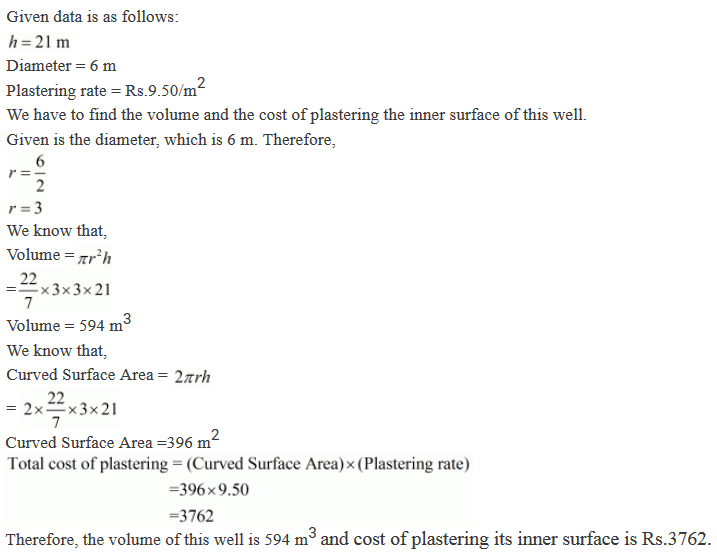19. The trunk of a tree is cylindrical and its circumference is 176 cm. If the length of the trunck is 3 m. Find the volume of the timber that can be obtained from the trunk.

Solution20. A well with 14 m diameter is dug 8 m deep. The earth taken out of it has been evenly spread all around it to a width of 21 m to form an embankment. Find the height of the embankment.

Solution21. The difference between inside and outside surfaces of a cylindrical tube 14 cm long is 88 sq. cm. If the volume of the tube is 176 cubic cm, find the inner and outer radii of the tube.

Solution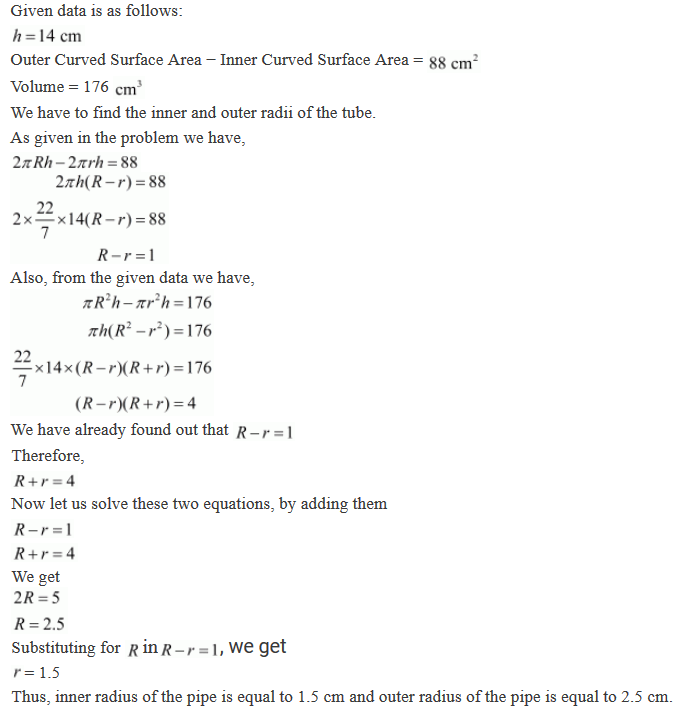22. Water flows out through a circular pipe whose internal diameter is 2 cm, at the rate of 6 metres per second into a cylindrical tank. The radius of whose base is 60 cm. Find the rise in the level of water in 30 minutes ?

Solution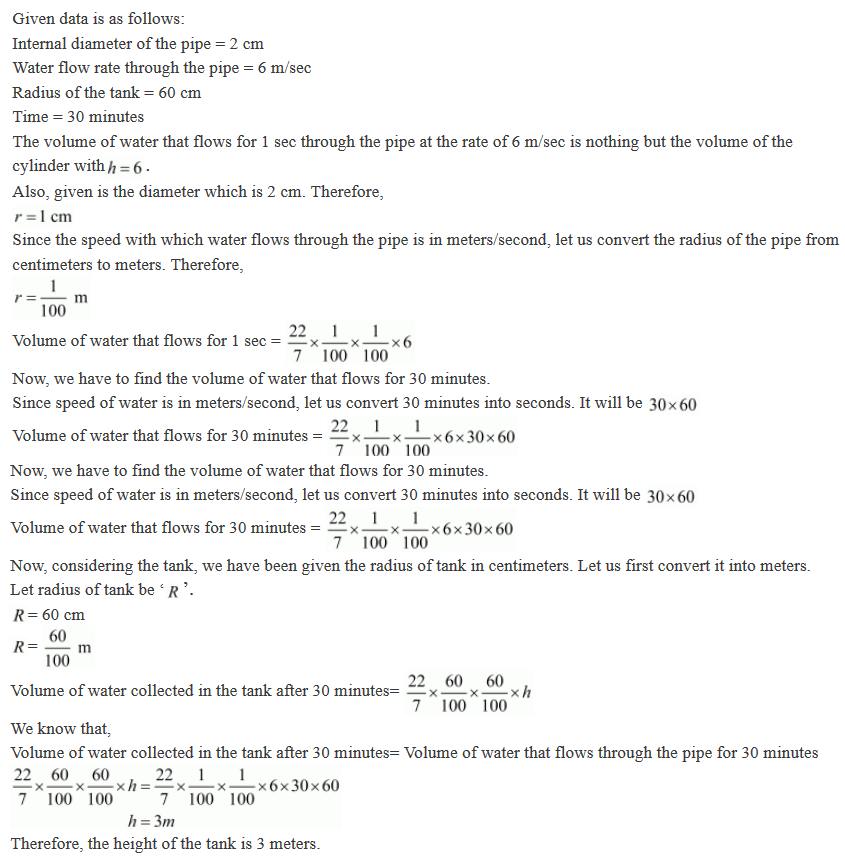23. A cylindrical container with diameter of base 56 cm contains sufficient water to submerge a rectangular solid of iron with dimensions 32 cm × 22 cm × 14 cm. Find the rise in the level of the water when the solid is completely submerged.

Solution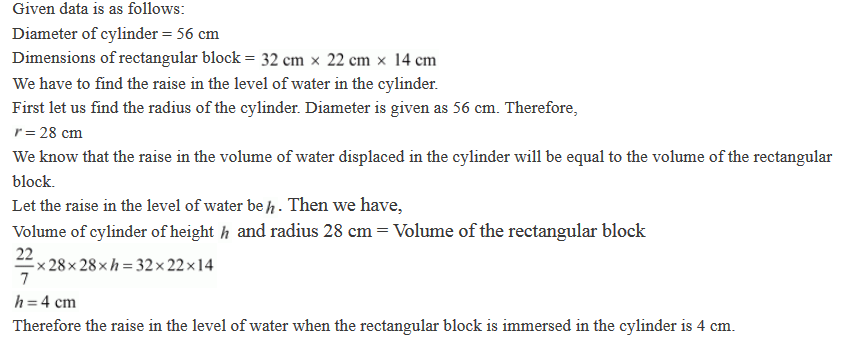24. A cylindrical tube, open at both ends, is made of metal. The internal diameter of the tube is 10.4 cm and its length is 25 cm. The thickness of the metal is 8 mm everywhere. Calculate the volume of the metal.

Solution25. From a tap of inner radius 0.75 cm, water flows at the rate of 7 m per second. Find the volume in litres of water delivered by the pipe in one hour.

Solution26. A cylindrical water tank of diameter 1.4 m and height 2.1 m is being fed by a pipe of diameter 3.5 cm through which water flows at the rate of 2 metre per second. In how much time the tank will be filled?

Solution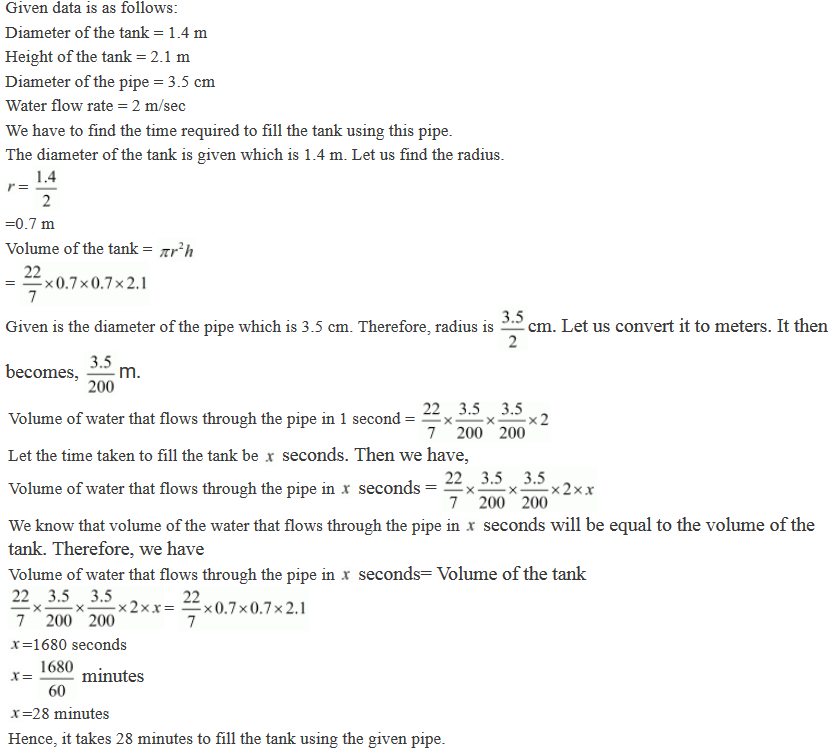27. A rectangular sheet of paper 30 cm × 18 cm can be transformed into the curved surfaced of a right circular cylinder in two ways i.e., either by rolling the paper along its length or by rolling it along its breadth. Find the ratio of the volumes of the two cylinders thus formed.

Solution28. How many litres of water flow out of a pipe having an area of cross-section of 5cm2 in one minute, if the speed of water in the pipe is 30 cm/ sec?

Solution29. The sum of the radius of the base and height of a solid cylinder is 37 m. If the total surface area of the solid cylinder is 1628 cm2. Find the volume of the cylinder.

Solution30. Find the cost of sinking a tubewell 280 m deep, having diameter 3 m at the rate of Rs 3.60 per cubic metre. Find also the cost of cementing its inner curved its inner curved surface at Rs 2.50 per square metre.

Solution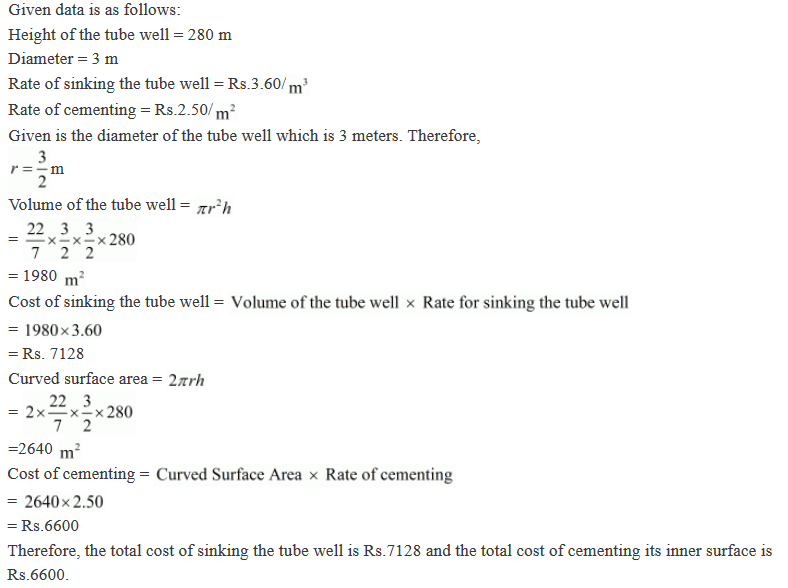31. Find the length of 13.2 kg of copper wire of diameter of 4 mm, when 1 cubic cm of copper weighs 8.4 gm .

Solution32. A well with 10 m inside diameter is dug 8.4 m deep . Earth taken out of it is spread all around it to a width of 7.5 m to form an embankment.Find the height of the embankment.

Solution